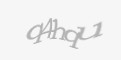# Solved - Arithmetic overflow error converting float to data type numericAre you trying convert float to numeric and you get "Arithmetic overflow error converting float to data type numeric" error? This could happen even if all was working before and now it fails. The reason should be in precision and scales settings.

For example we had some calc formula for FX rates, Cast(number1 - number2 as Numeric(18 ,18), all was working fine, until one time when Arithmetic overflow error appears.

To find reason and solution lets take a look at NUMERIC function and parameters.

NUMERIC(p, s) function has parameters p - precision, s - scale

Important part is that precision parameter means both left and right decimal. scale is on right decimal

For example:

Number 12.532 has precision = 5, scale = 3, if we will try use conversion to Numeric(3,3) it will fails, but Numeric(5,3) will work in this case.

Which cause error "Arithmetic overflow error converting numeric to data type numeric." for this code

`SELECT Cast(12.532 as Numeric(3, 3)) as Number`

Working for this code

`SELECT Cast(12.532 as Numeric(5, 3)) as Number`

If you get error, take a look at numbers, check how many decimal places you have on both sides and how many on right side from decimal point and fix NUMERIC function parameters. Most probably increase precision, or lower scale.

It is more likely to happen, when you have very small numbers with many decimal places combine with some big numbers that also use many decimal places.

Details about NUMERIC function you can find on Microsoft site https://docs.microsoft.com/en-us/sql/t-sql/data-types/decimal-and-numeric-transact-sql

Does it help to you? Feel free to share and comment.

Author info
Author: Stanislav DubenWebsite: http://www.duben.org